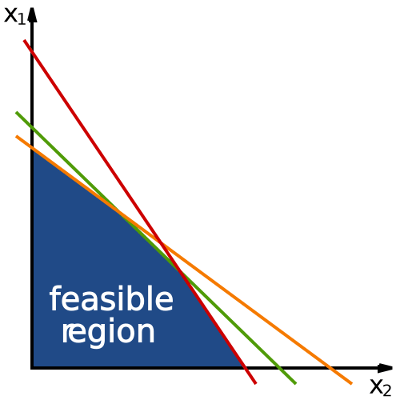In one of my projects I need to solve a Linear Programming problem and I found LPSolve, a Linear Programming library that fits my requirements. Unfortunately the library is written in C so I created a LPSolve NodeJS NPM module

Thanks to nan you can create a wrapper for your C libraries in NodeJS. I’ll post a tutorial to do it

At this moment the module is in Alpha-1. There are a lot of methods that are NOT implemented yet

LPSolve NodeJS Module Usage

If you want to solve a Linear Programming with this module you can do it reading a LP file directly

```'use strict'

var NodeSolve = require('nodesolve')

var problem = NodeSolve.readLP('problem.lp', NodeSolve.VERBOSITY.NORMAL, 'problem')

problem.solve(function (err, status) { // Solve problem asynchronously
var variables = problem.variables()
console.log(variables)
})
```

Or you can create your own using the Nodesolve API

```'use strict'

var NodeSolve = require('nodesolve')

var problem = new NodeSolve()

problem.resize(1, 2)
problem.objective([1, 1])
problem.maxim(false)

problem.intVar(0, true)
problem.lowBound(0, 1)
problem.lowBound(1, 1)

problem.constraint([1, 1], NodeSolve.CONSTRAINT_TYPE.GE, 2)

var status = problem.solve() // Solve problem synchronously
var variables = problem.variables()
```

If you need more examples you can check out the jsdoc of the project under the doc folder or you can check out the tests of the module

The module is in continuous development so stay tuned. You can star, watch or fork the repository

comentarios